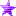# Outerra forum

• February 23, 2020, 03:41:29 pm• Welcome, Guest

Pages: 

###AuthorTopic: Airplane texture and windscreen allways show snow-flakes  (Read 501 times)

#### fly77

• Hero Member
•• Posts: 684##### Airplane texture and windscreen allways show snow-flakes
« on: March 19, 2019, 05:13:47 pm »

After having fiddled with snowlevel (lowering it) I now have allways snowflakes on windows of airplanes and mirrors of some vehicles and the mig-29, even if I set them back to higher values. Its very annoying. How to set back to normal the screens ? here are my eng.cfg settings and here my eng.log  file   https://www.dropbox.com/s/aj9que0au00dimb/eng.log?dl=0

Code: [Select]
`num_jobproc_threads = 1,renderer_config = { screen_width = 2560, screen_height = 1080, fullscreen = false, swap_interval = 0, msaa = 1, debug_opengl = false, debug_async = true, refresh_rate = 60},videos_dir = "C:/Users/novelchip/Outerra\videos",screenshots_dir = "C:/Users/novelchip/Outerra\screenshots",terrain_shadows = true,tree_shadows = true,wnd_x_pos = 0,wnd_y_pos = 0,wnd_hide_titlebar = true,mouse_exclusive = false,sim_mode = false,fov = 83.599998,fov_v_separate = 0.0,proj = 0,enable_hid_logging = false,enable_jsbsim_logging = false,mouse_sensitivity = 1.0,joy_sensitivity = 1.0,mouse_invert = false,flightmode_invert = true,pause_in_sandbox = true,debug_keys = false,cfg-version = 10447,bloom_power = 0.03,bloom_threshold = 1.0,min_obj_size = 5.0,sound = { global = 1.0, ambient = 1.0, vehicle = 0.5, effect = 0.09, music = 1.0, hrtf = true},use_hmd = false,video = 1,ssao_quality = 2,shadow_quality = 2,stereo = { eye_separation = 0.064, convergence = 0.1, reduce_grass = false, force_hrtf = true, stereo_type = 0, swap_sides = false},controller = { pan_min_angle = { x = -1.570796, y = -0.785398 }, pan_max_angle = { x = 1.570796, y = 0.785398 }, panning_speed = { x = 0.01, y = 0.01 }, view_min_angle = { x = -1.570796, y = -0.785398 }, view_max_angle = { x = 1.570796, y = 0.785398 }, view_delay = 0.1, fm_roll_coef = 0.4, fm_turn_coef = 1.25, fm_turn_delay = 4.0, fm_filter_delay = 0.5, um_filter_delay = 0.02, hmd_turn_coef = 1.0, hmd_pitch_coef = 0.2, vr_ufo_pitch = false, vr_mouse_pitch = false, vr_ufo_ref = 2, vr_fps_ref = 3},perf_report = { enabled = false, sync = false, ui = 5.0, scene = 5.0, world = 5.0, ext = 5.0, rungc = 0},pkg_limits = { max_meshes = 4},disable_log_popup = false,img_cache_slice_size = 2048,img_cache_num_slices = 2,loglevel = "info"`
« Last Edit: March 19, 2019, 05:57:52 pm by fly77 »Logged

#### fly77

• Hero Member
•• Posts: 684##### Re: Airplane texture and windscreen allways show snow-flakes
« Reply #1 on: March 20, 2019, 03:21:09 am »

I did ALT+F8 as suggested by KW71 on another post and the problem disappear. Thanks to KW71.Logged
Pages: